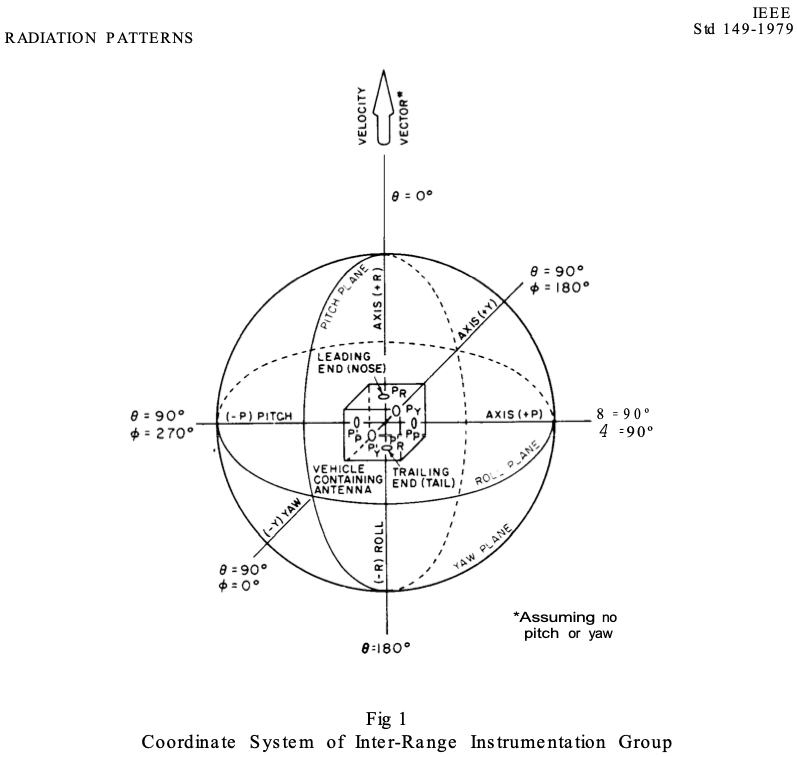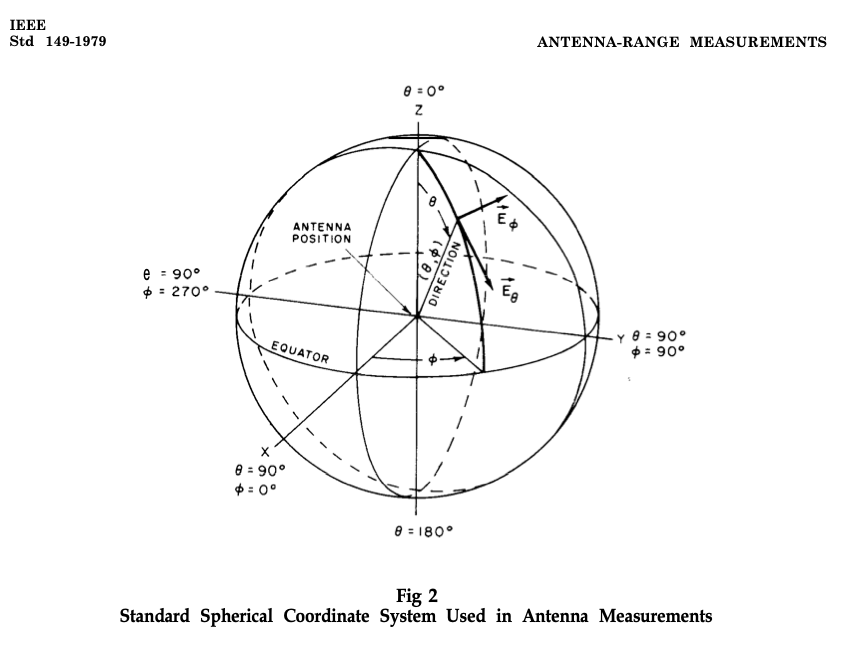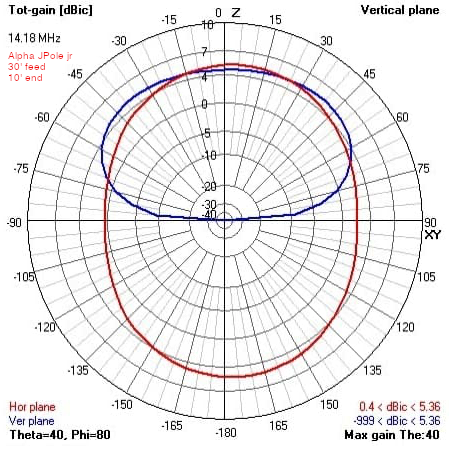• Below are two diagrams illustrating the IEEE coordinate system for antenna field strength measurement.
• Note that nowhere in either is the orientation of the antenna considered.  To analyze this antenna, I will assume the antenna lies from X=0,Y=0,Z=0 at the feed point to X=34,Y=-32.5,Z=-20.  The interior angle of the radiator to horizontal is 36 degrees.
••• Below is the 20M radiation pattern of the JPole jr provided by Alpha.
• NOTE: dBic is decibels gain referenced to a circularly polarized, theoretical isotropic radiator.
• In the horizontal plane then maximum gain is 5.36 dBic which occurs at +5 and +172 degrees.  Given the installed orientation is feed point North radiator South then actual gain angles would be 352 True and 175 True.
• In the vertical plane the maximum gain is 5.36 dBic at -40 and +40 degrees (Theta) relative to the end of the radiator, at a vertical angle (Phi) of 80 degrees.
• NOTE: If the antenna was analyzed as an isotropic radiator (no reference to a ground plane) then the 36 degree slope would subtract from the 80 degrees giving a departure angle of 44 degrees toward the South Southwest and South Southeast with only minor power change between 125 True and 235 True.
• NOTE: If you look at some of my 'signal reports' screen captures from PSKReporter you will see that this setup prefers Southern California, and an arc across North America from North of Chicago to The Gulf of Mexico on 20M nearly anytime.  Only occasionally does it get signal into Central and South America, but Hawaii, New Zealand, Australia and Japan are regular openings on 20M after sunset.